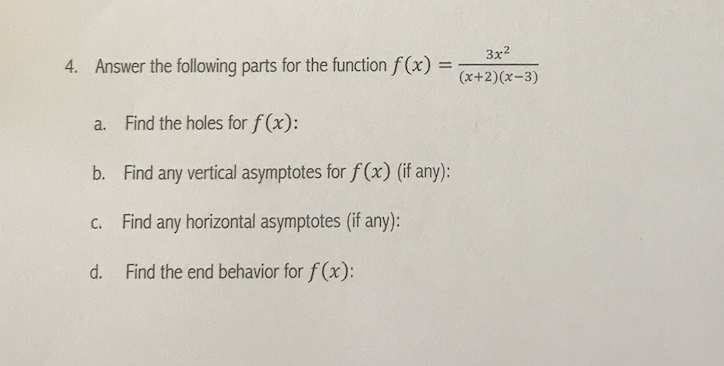# 3x2Answer the following parts for the function f(x) =x+ 2 ) ( x - 3 )4.Find the holes for f(x):a.Find any vertical asymptotes for f(x) (if any):b.Find any horizontal asymptotes (if any):c.Find the end behavior for f(x):d.

Question
1 viewshelp_outlineImage Transcriptionclose3x2 Answer the following parts for the function f(x) =x+ 2 ) ( x - 3 ) 4. Find the holes for f(x): a. Find any vertical asymptotes for f(x) (if any): b. Find any horizontal asymptotes (if any): c. Find the end behavior for f(x): d. fullscreen
check_circle

Step 1

Since we only answer up to 3 sub-parts, we’ll answer the first 3. Please resubmit the question and specify the other subparts (up to 3) you’d like answered

Step 2

Given,

Step 3

Part (a):

In order to find, holes of function,

Check, any common factor of numerator and denominator exist or not.

Case I: If common fac...

### Want to see the full answer?

See Solution

#### Want to see this answer and more?

Solutions are written by subject experts who are available 24/7. Questions are typically answered within 1 hour.*

See Solution
*Response times may vary by subject and question.
Tagged in

### Functions